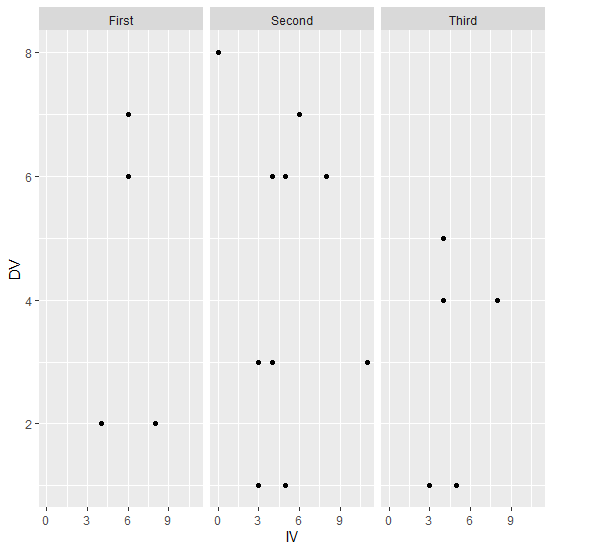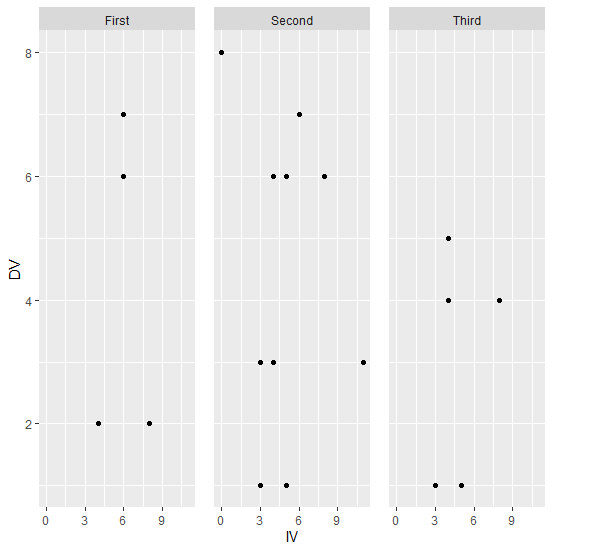# Increase the space between facets in a facetted plot created using ggplot2 in R.

R ProgrammingServer Side ProgrammingProgramming

#### Artificial Intelligence : The Future Of Programming

15 Lectures 54 mins

#### Beyond Basic Programming - Intermediate Python

Most Popular

36 Lectures 3 hours

#### C Programming from scratch- Master C Programming

Best Seller

60 Lectures 8 hours

To increase the space between facets in a facetted plot created by using ggplot2 in R, we can use theme function with panel.spacing argument.

For Example, if we have a data frame called df that contains three columns say X, Y and F where F is a factor column then we can create facetted scatterplots having facets spaced at a larger distance between X and Y for values in F by using the command given below −

ggplot(df,aes(X,Y))+geom_point()+facet_wrap(~F)+theme(panel.spacing=unit(1,"lines"))

## Example

Following snippet creates a sample data frame −

IV<-rpois(20,5)
DV<-rpois(20,5)
Group<-sample(c("First","Second","Third"),20,replace=TRUE)
df<-data.frame(IV,DV,Group)
df

The following dataframe is created

  IV DV Group
1 4  3 Second
2 5  6 Second
3 3  1 Second
4 4  4 Third
5 5  1 Third
6 3  3 Second
7 4  3 Second
8 4  6 Second
9 8  6 Second
10 0  8 Second
11 4  2 First
12 4  5 Third
13 5  1 Second
14 8  4 Third
15 3  1 Third
16 8  2 First
17 6  7 First
18 6  7 Second
19 11 3 Second
20 6  6 First

To load ggplot2 package and create facetted scatterplot between IV and DV with facets based on values in Group column −

IV<-rpois(20,5)
DV<-rpois(20,5)
Group<-sample(c("First","Second","Third"),20,replace=TRUE)
df<-data.frame(IV,DV,Group)
library(ggplot2)
ggplot(df,aes(IV,DV))+geom_point()+facet_wrap(~Group)

## Output

If you execute all the above given snippets as a single program, it generates the following Output −To create facetted scatterplot between IV and DV with facets spaced at a larger distance based on values in Group column on the above created data frame, add the following code to the above snippet −

IV<-rpois(20,5)
DV<-rpois(20,5)
Group<-sample(c("First","Second","Third"),20,replace=TRUE)
df<-data.frame(IV,DV,Group)
library(ggplot2)
ggplot(df,aes(IV,DV))+geom_point()+facet_wrap(~Group)+theme(panel.spacing=unit(1,"lines"))

## Output

If you execute all the above given snippets as a single program, it generates the following Output −